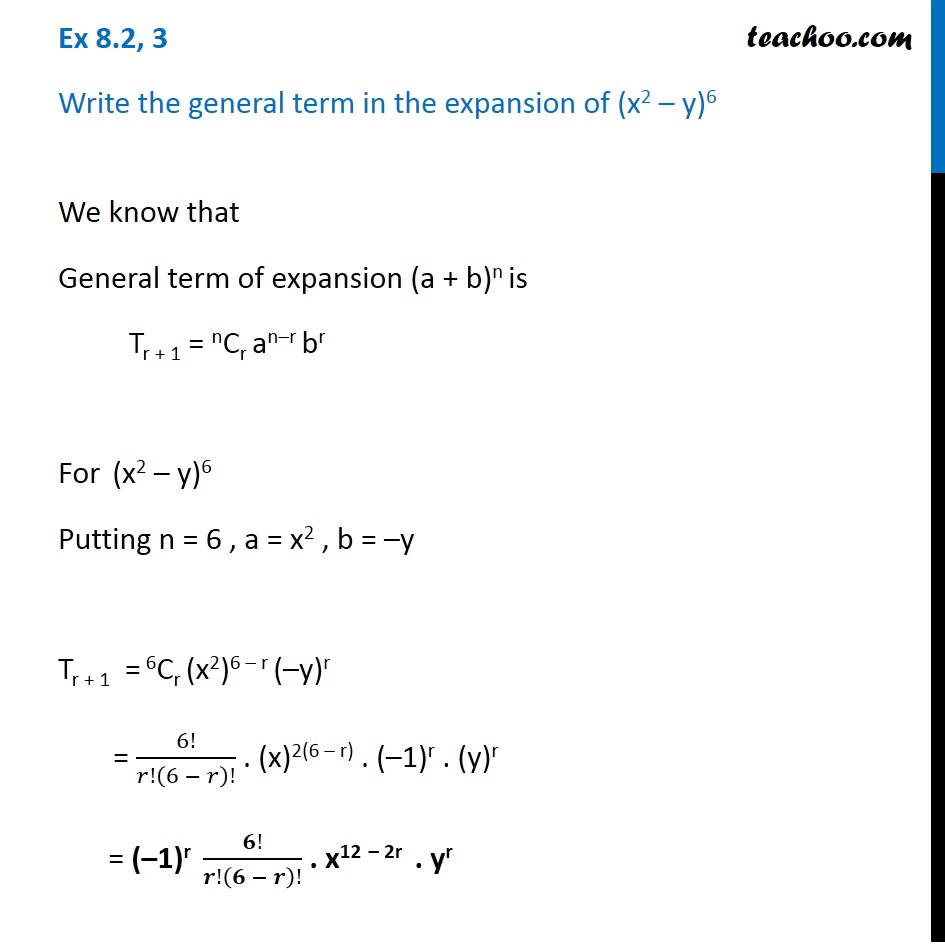General Term - Defination

Chapter 8 Class 11 Binomial Theorem (Deleted)
Concept wise### Transcript

Ex 8.2, 3 Write the general term in the expansion of (x2 – y)6 We know that General term of expansion (a + b)n is Tr + 1 = nCr an–r br For (x2 – y)6 Putting n = 6 , a = x2 , b = –y Tr + 1 = 6Cr (x2)6 – r (–y)r = 6!/𝑟!(6 − 𝑟)! . (x)2(6 – r) . (–1)r . (y)r = (–1)r 𝟔!/𝒓!(𝟔 − 𝒓)! . x12 – 2r . yr